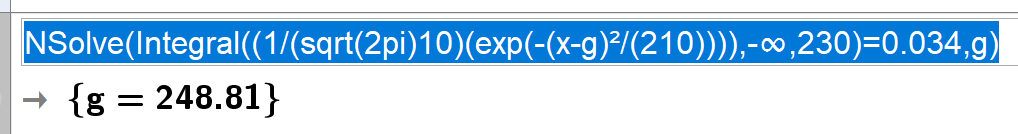# gauss function

ismailiblearti shared this question 1 year ago

can someone help me (int from -inf to 230 (1/(sqrt(2pi)10)(e^((x-g)²/(210)))))=0.034 how can i get the average (g) out of this its the gauss function1

You're missing a minus sign. Try this:

```NSolve(Integral((1/(sqrt(2pi)10)(exp(-(x-g)²/(210)))),-∞,230)=0.034,g)
```